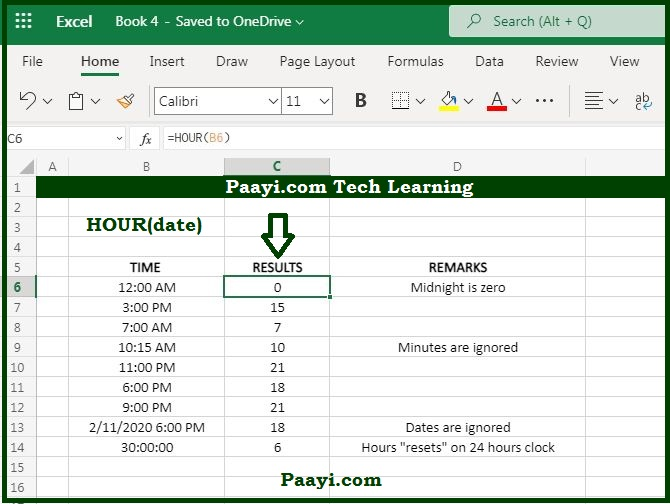# Learn How to Use Microsoft Excel HOUR Function

Written by | 0 Comments | 722 Views

In this article, you will learn how to use the MS Excel HOUR function and its prime function in Microsoft Excel. You will also get to know the Microsoft Excel HOUR function return value and syntax with the help of some examples.

## Microsoft Excel Hour Function

The main purpose of the HOUR function is to get an hour as a number (0-23) from the time. That means you can use the MS Excel HOUR function to return the hour component of the time as a number between 0 - 23. For example, with the given time 8:30 AM, the HOUR function will return 8, so the HOUR function can be used to extract hour into the particular cell, similar to the TIME function.

### HOUR Function Return Value

HOUR function will return the number between 0 to 23.

### HOUR Function Syntax

= HOUR(serial-number)

Where:

• serial-number: It is the valid Excel time.

## How to Use Microsoft Excel HOUR Function?The HOUR function will return the hour from the specified time, you can understand by the examples given below:

Example 1: = HOUR("10:00 AM") - The return value will be 10.

Example 2: = HOUR("10:00 PM") - The return value will be 22.

You can also use the HOUR function to extract a time into a specified cell or feed a result into another formula. Let's say; for example, the time is "9:00 AM" is A1, you can change the time with 30 minutes like:

Example 3: = TIME(HOUR(A1),30,0) - This will return 9:30 AM.